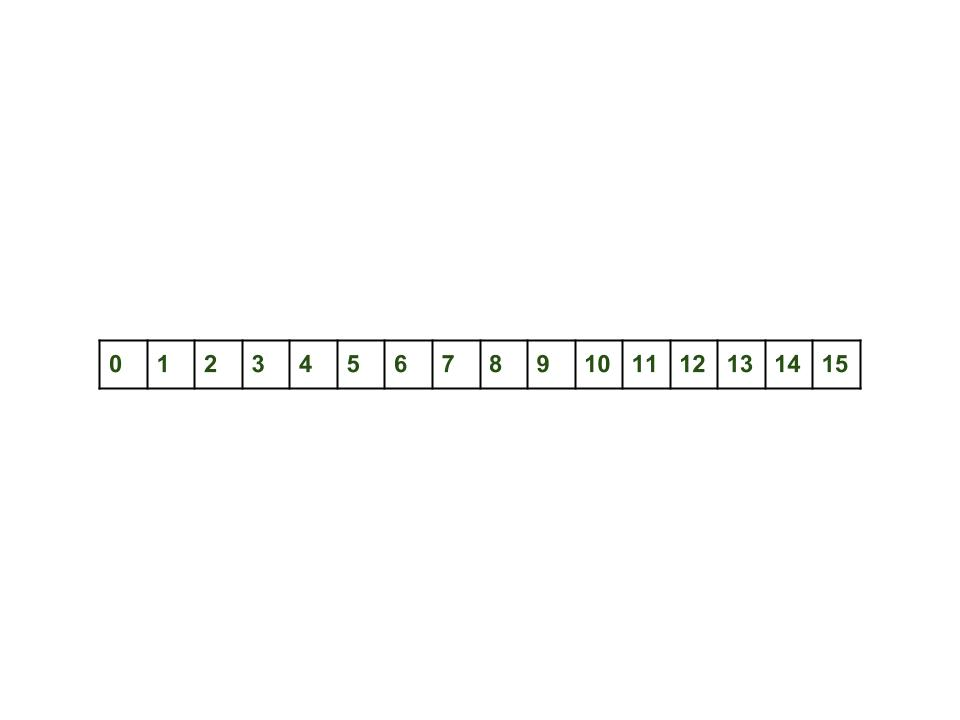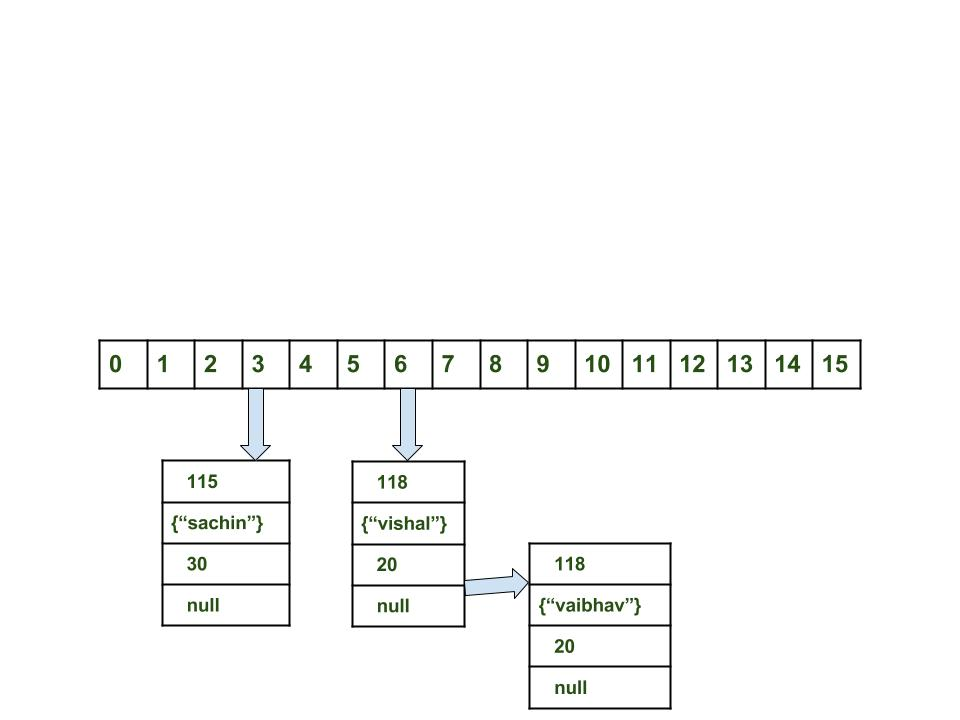# Internal Working of HashMap in Java

• Difficulty Level : Medium
• Last Updated : 31 Dec, 2021

In this article, we will see how hashmap’s get and put method works internally. What operations are performed. How the hashing is done. How the value is fetched by key. How the key-value pair is stored.
As in previous article, HashMap contains an array of Node and Node can represent a class having following objects :

1. int hash
2. K key
3. V value
4. Node next

Now we will see how this works. First we will see the hashing process.

Hashing

Hashing is a process of converting an object into integer form by using the method hashCode(). Its necessary to write hashCode() method properly for better performance of HashMap. Here I am taking key of my own class so that I can override hashCode() method to show different scenarios. My Key class is

```//custom Key class to override hashCode()
// and equals() method
class Key
{
String key;
Key(String key)
{
this.key = key;
}

@Override
public int hashCode()
{
return (int)key.charAt(0);
}

@Override
public boolean equals(Object obj)
{
return key.equals((String)obj);
}
}```

Here overrided hashCode() method returns the first character’s ASCII value as hash code. So whenever the first character of key is same, the hash code will be same. You should not approach this criteria in your program. It is just for demo purpose. As HashMap also allows null key, so hash code of null will always be 0.

hashCode() method

hashCode() method is used to get the hash Code of an object. hashCode() method of object class returns the memory reference of object in integer form. Definition of hashCode() method is public native hashCode(). It indicates the implementation of hashCode() is native because there is not any direct method in java to fetch the reference of object. It is possible to provide your own implementation of hashCode().
In HashMap, hashCode() is used to calculate the bucket and therefore calculate the index.

equals() method

equals method is used to check that 2 objects are equal or not. This method is provided by Object class. You can override this in your class to provide your own implementation.
HashMap uses equals() to compare the key whether they are equal or not. If equals() method return true, they are equal otherwise not equal.

Buckets

A bucket is one element of HashMap array. It is used to store nodes. Two or more nodes can have the same bucket. In that case link list structure is used to connect the nodes. Buckets are different in capacity. A relation between bucket and capacity is as follows:

`capacity = number of buckets * load factor`

A single bucket can have more than one nodes, it depends on hashCode() method. The better your hashCode() method is, the better your buckets will be utilized.

Index Calculation in Hashmap

Hash code of key may be large enough to create an array. hash code generated may be in the range of integer and if we create arrays for such a range, then it will easily cause outOfMemoryException. So we generate index to minimize the size of array. Basically following operation is performed to calculate index.

`index = hashCode(key) & (n-1).`

where n is number of buckets or the size of array. In our example, I will consider n as default size that is 16.

Why the above method is used to calculate the index

Using a bitwise AND operator is similar to doing bit masking wherein only the lower bits of the hash integer are considered which in turn provides a very efficient method of calculating the modulus based on the length of the hashmap.

• Initially Empty hashMap: Here, the hashmap is size is taken as 16.

`HashMap map = new HashMap();`
• HashMap :• Inserting Key-Value Pair: Putting one key-value pair in above HashMap

`map.put(new Key("vishal"), 20);`
• Steps:
1. Calculate hash code of Key {“vishal”}. It will be generated as 118.
2. Calculate index by using index method it will be 6.
3. Create a node object as :

```{
int hash = 118

// {"vishal"} is not a string but
// an object of class Key
Key key = {"vishal"}

Integer value = 20
Node next = null
}```
1.
2. Place this object at index 6, if no other object is presented there.
• Inserting another Key-Value Pair: Now, putting other pair that is,

`map.put(new Key("sachin"), 30);`
• Steps:
1. Calculate hashCode of Key {“sachin”}. It will be generated as 115.
2. Calculate index by using index method it will be 3.
3. Create a node object as :

```{
int hash = 115
Key key = {"sachin"}
Integer value = 30
Node next = null
}```
•

• In Case of collision: Now, putting another pair that is,

`map.put(new Key("vaibhav"), 40);`
• Steps:
1. Calculate hash code of Key {“vaibhav”}. It will be generated as 118.
2. Calculate index by using index method it will be 6.
3. Create a node object as :
``` {
int hash = 118
Key key = {"vaibhav"}
Integer value = 40
Node next = null
}```
1. Place this object at index 6 if no other object is presented there.
2. In this case a node object is found at the index 6 – this is a case of collision.
3. In that case, check via hashCode() and equals() method that if both the keys are same.
4. If keys are same, replace the value with current value.
5. Otherwise connect this node object to the previous node object via linked list and both are stored at index 6.
Now HashMap becomes :Using get method()

Now lets try some get method to get a value. get(K key) method is used to get a value by its key. If you don’t know the key then it is not possible to fetch a value.

• Fetch the data for key sachin:
`map.get(new Key("sachin"));`
• Steps:
1. Calculate hash code of Key {“sachin”}. It will be generated as 115.
2. Calculate index by using index method it will be 3.
3. Go to index 3 of array and compare first element’s key with given key. If both are equals then return the value, otherwise check for next element if it exists.
4. In our case it is found as first element and returned value is 30.
• Fetch the data for key vaibahv:

`map.get(new Key("vaibhav"));`
• Steps:
1. Calculate hash code of Key {“vaibhav”}. It will be generated as 118.
2. Calculate index by using index method it will be 6.
3. Go to index 6 of array and compare first element’s key with given key. If both are equals then return the value, otherwise check for next element if it exists.
4. In our case it is not found as first element and next of node object is not null.
5. If next of node is null then return null.
6. If next of node is not null traverse to the second element and repeat the process 3 until key is not found or next is not null.
7. Time complexity is almost constant for put and get method until rehashing is not done.
8. In case of collision, i.e. index of two or more nodes are same, nodes are joined by link list i.e. second node is referenced by first node and third by second and so on.
9. If key given already exist in HashMap, the value is replaced with new value.
10. hash code of null key is 0.
11. When getting an object with its key, the linked list is traversed until the key matches or null is found on next field.
My Personal Notes arrow_drop_up